JEE  >  NCERT Solutions Exercise 5.6: Continuity & Differentiability

# NCERT Solutions Exercise 5.6: Continuity & Differentiability | Mathematics For JEE

 1 Crore+ students have signed up on EduRev. Have you?

Continuity & Differentiability

Question 1:
If x and y are connected parametrically by the equation, without eliminating the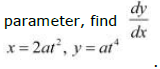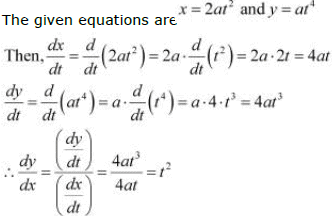Question 2: If x and y are connected parametrically by the equation, without eliminating the
parameter, find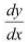.
x = a cos θ, y = b cos θ

The given equations are x = a cos θ and y = b cos θ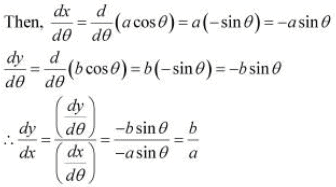Question 3: If x and y are connected parametrically by the equation, without eliminating the
parameter, find.
x = sin t, y = cos 2t

The given equations are x = sin t and y = cos 2t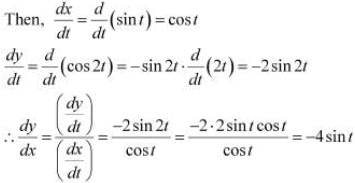Question 4:
If x and y are connected parametrically by the equation, without eliminating the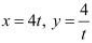.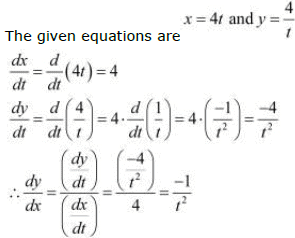Question 5: If x and y are connected parametrically by the equation, without eliminating the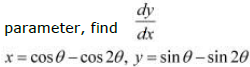The given equations are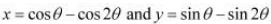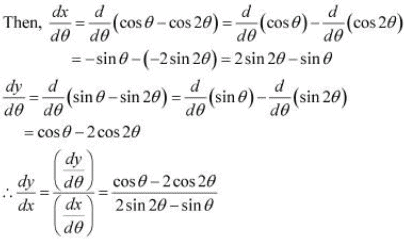Question 6: If x and y are connected parametrically by the equation, without eliminating the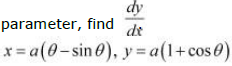The given equations are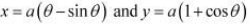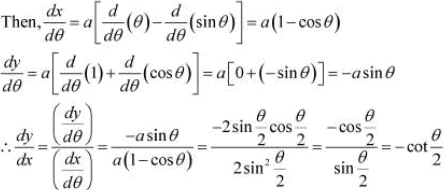Question 7: If x and y are connected parametrically by the equation, without eliminating the

parameter,  find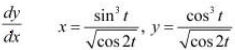.

The given equations are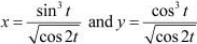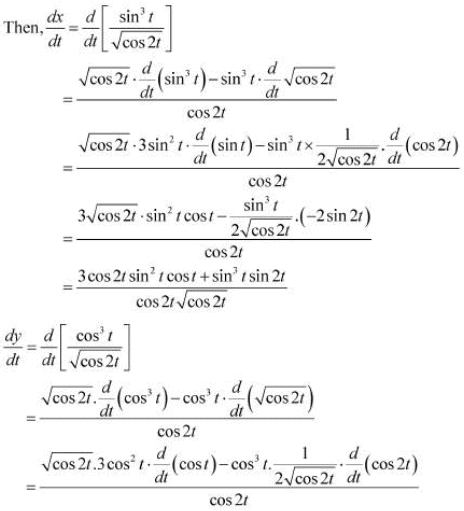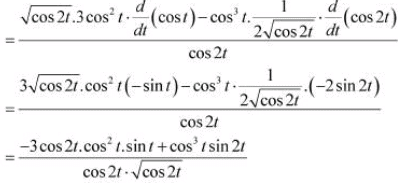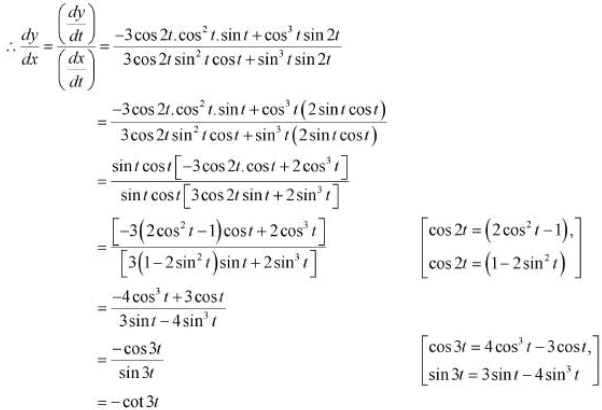Question 8: If x and y are connected parametrically by the equation, without eliminating the

parameter, find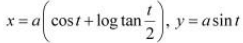The given equations are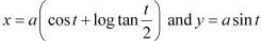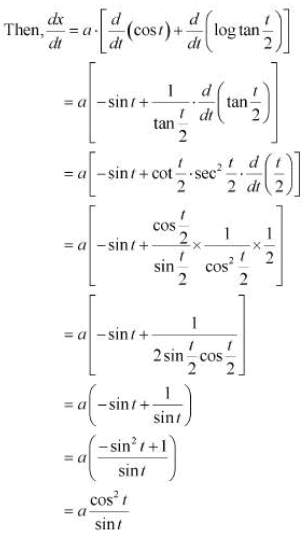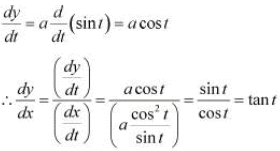Question 9: If x and y are connected parametrically by the equation, without eliminating the
parameter, find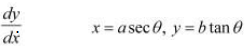.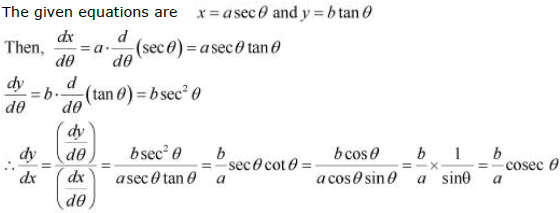Question 10: If x and y are connected parametrically by the equation, without eliminating the

parameter, find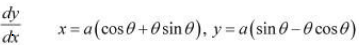.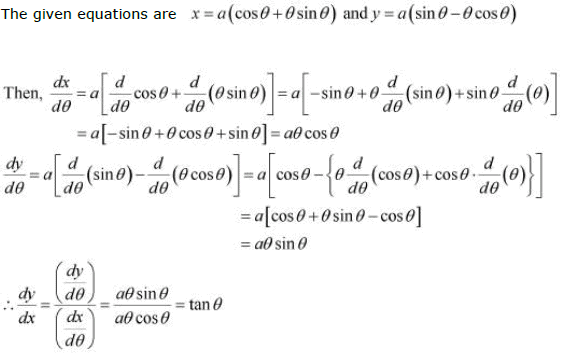Question 11:
If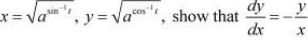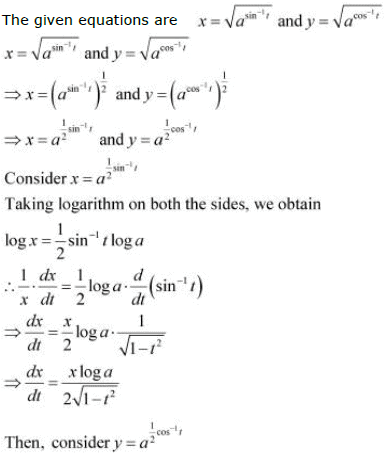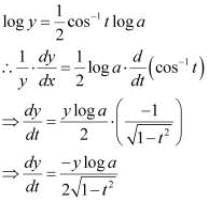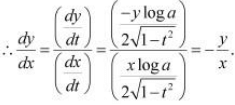Hence, proved.

The document NCERT Solutions Exercise 5.6: Continuity & Differentiability | Mathematics For JEE is a part of the JEE Course Mathematics For JEE.
All you need of JEE at this link: JEE

## Mathematics For JEE

130 videos|359 docs|306 tests

## Mathematics For JEE

130 videos|359 docs|306 tests

Track your progress, build streaks, highlight & save important lessons and more!(Scan QR code)

,

,

,

,

,

,

,

,

,

,

,

,

,

,

,

,

,

,

,

,

,

;